# Test: Rational Numbers

## 20 Questions MCQ Test NCERT Mathematics for CAT Preparation | Test: Rational Numbers

Description
Attempt Test: Rational Numbers | 20 questions in 20 minutes | Mock test for Class 9 preparation | Free important questions MCQ to study NCERT Mathematics for CAT Preparation for Class 9 Exam | Download free PDF with solutions
QUESTION: 1

### If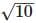= 3.162, then the value of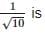Solution: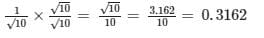QUESTION: 2

### Which of the following is the product of 7/8 and -4/21?

Solution:

7/8 x -4/21 = -1/6

Option B is the correct answer.

QUESTION: 3

### Rationalise the denominator of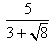.

Solution: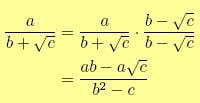5 / (3 + √8)  x  ( (3 - √8) /  (3 - √8)

=  5 ( 3 - √8)/(32 - 8 )

= 5 ( 3 - √8 ) / 9 - 8  = 15 - 5√8

So option C is correct.

QUESTION: 4

Expression of 2.2323… in the form of a/ b is ________.

Solution:
QUESTION: 5

The rational number between 1 and 2 is

Solution:

Remember the general formula to find rational number between two

given number 1/2(a+b)[where a, b are given numbers]

A rational number between 1 and 2 is 1/2(1+2)=3/2

QUESTION: 6

The sum of the digits of a number is subtracted from the number, the resulting number is always divisible by:

Solution:

Let the three digit number be 439
The sum of digits =16
Difference =439−16=423 which is divisible by 9.

QUESTION: 7

Which of the following lies between 0 and -1?

Solution:
QUESTION: 8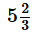is

Solution:
QUESTION: 9

p/q is a rational number, so p and q must be

Solution:
QUESTION: 10

Which of the following is the product of 7/8 and -4/21?

Solution:

7 / 8 x -4 / 21 = -1/6

So answer is option A .

QUESTION: 11

From the choices given below mark the co-prime numbers

Solution:
QUESTION: 12

All the integers are

Solution:

The rational numbers include all the integers, plus all fractions, or terminating decimals and repeating decimals. Every rational number can be written as a fraction a/b, where aand b are integers. For example, 3 can be written as 3/1, -0.175 can be written as -7/40, and 1 1/6 can be written as 7/6. All natural numbers, whole numbers, and integers are rationals, but not all rational numbers are natural numbers, whole numbers, or integers.

QUESTION: 13

The fraction equivalent of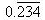is

Solution:

Let x = 0.234234234.........
(1) Then , multiply both side with 1000 1000x = 234.234234......(2) Now Eq.(2)-(1) 1000x-x = 234.234234- 0.234234 999x = 234 x = 234/999

QUESTION: 14

Between 3 and 4 there are

Solution:

There is no single number between 3 and 4, there is an infinite amount of numbers. If you are asking for INTEGERS, there are none. If not, you can have unlimited numbers between 3 and 4. For example, a number could be 3.0000000000000000005 or 3.9999999999999997.

QUESTION: 15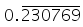in the form of fraction is

Solution:

X=0.230769
(1) 1000000=230769.230769

(2) subtracting

(1) from (2) 230769 =9999999x then, x =230769/9999999 =3/13

QUESTION: 16

Every rational number is

Solution:

Real numbers are the numbers that can be placed on number line
And rational number can be placed on number line so all rational numbers are real number.

QUESTION: 17

The product of two numbers is -20/9. If one of the numbers is 4, find the other.

Solution:
QUESTION: 18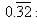in the form of a fraction is

Solution: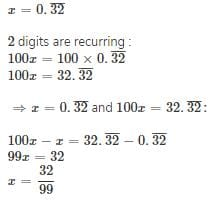QUESTION: 19

If  5/13 = 0.384615……, then the value of 10/13 _____

Solution:
QUESTION: 20

What should be added to -5/4 to get -1?

Solution:

- 5 / 4 + x = -1

x = -1 + 5 / 4

x = (-4 + 5) / 4

x = 1 / 4Use Code STAYHOME200 and get INR 200 additional OFF Use Coupon Code